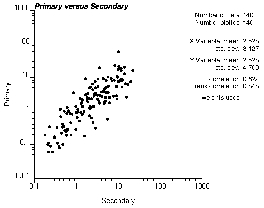GSLIB Help Page: SCATPLTDescription:
• scatplt displays bivariate scatterplots and some statistical summaries for PostScript display. The summary statistics are weighted, except for the rank order (Spearman) correlation coefficient.Parameters:
• datafl: The data file in a simplified Geo-EAS format.
• icolx, icoly, icolwt and icol3vr: The columns for the x variable (horizontal axis), y variable (vertical axis), weight (if less than or equal to zero then equal weighting is used; otherwise, the weight is used to calculate the summary statistics), and third variable (if less than or equal to zero then no third variable is considered; otherwise, each bullet is gray scale coded according to this third variable)
• tmin and tmax: all values strictly less than tmin and strictly greater than tmax are ignored.
• outfl: file for PostScript output.
• xmin, xmax and xlog: minimum and maximum plotting limits for the x axis. These do not have to be compatible with the trimming limits. Pairs within the trimming limits, but outside the plotting limits, are still used for computing the correlation coefficient. Setting xmin greater than or equal to xmax will cause the program to use the minimum and maximum data values encountered. If xlog=0 then an arithmetic scaling of the x axis is used, xlog=1 then a logarithmic scaling is used.
• ymin, ymax and ylog: minimum and maximum plotting limits for the y axis. Setting ymin greater than or equal to ymax will cause the program to use the minimum and maximum data values encountered. If ylog=0 then an arithmetic scaling of the y axis will be used, ylog=1 then a logarithmic scaling will be used.
• nthpoint: plot every nthpoint'th data point. This should normally be set to 1, however, it may be used to subset large data sets.
• dotsiz: size of the black dot marking each data (0.1 is small, 1.0 is the default, and 10 is big)
• gmin and gmax: minimum and maximum gray scale limits: used if a third variable is considered to shade each data point
• title: a 40-character title for the top of the plot. A short description of the programApplication notes:
• If the scatterplot appears too erratic because of too few data, one may consider smoothing.
• The plot may become quite crowded if there are too many data pairs.